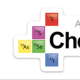# Which R do I use?March 09, 2013

NOTE: There is now an updated (April 2016) version of this post to reflect the new curriculum.

Which R do I use? It’s a common question for chemistry students, and when it comes to the AP chemistry exam there is a choice of values for the Universal Gas Constant. Currently appearing on an AP formula sheet near you, you can find the following;

a. R = 8.31 J mol-1 K-1

b. R = 0.0821 L atm mol-1 K-1

c. R = 8.31 volt coulomb mol-1 K-1

So which value do we use in which situation?

There are a couple of ways to tackle this. Firstly a student could simply learn which value to use in which situation, by simply memorizing the correct pairings. For example, when using P V = n R T where the pressure is given in atm and the volume in L, then use b. When applying the formula to calculate the root mean square speed of a gas, use a. Of course, this memorization method is not necessarily the preferable way to make sure that you are using the right R, since it does not involve any understanding as such, but having said that, if the choice is ‘learn the pairings parrot fashion with no understanding’, or not learn them at all, then I choose the former over the latter every time!

A ‘better’ way to handle the choice of R is to think about the units in each scenario. Below are a few common situations that should help students clarify which R is in play.

1. Applying P V = n R T with ideal gases. As long as the pressure is in atmospheres and the volume in L, then you use b. since it is the only one with L and atm in its units. There ARE other values for the gas constant that take into account other units of pressure like 62.4 L mmHg mol-1 K-1 for example, but since they are not on the current data packet, they are not in play. If you’d like to see a few more, click here.

2. Applying urms = SQRT (3RT/M) when calculating the root mean square speed of a gas. This is trickier, since you really have to know that a Joule = kg m2 s-2 (in SI base units) which means that an R that includes J will introduce meters per second (speed) when square rooted. As such, use a. (Joules as kg m2 s-2 also helps justify the use of units of kg in the denominator.

Given this kind of unit manipulation is ore associated with a physics course (not a chemistry course) in my opinion, this maybe a good situation and R pairing to simply learn and remember.

3. Applying ∆Go = – RT ln K and ∆G = ∆Go + RT ln Q when calculating Gibbs Free Energy. Not too difficult if you associate Gibbs free Energy with energy(!), and that therefore we must be dealing with Joules. Use a.

4. Using the Nernst equation, Ecell = Eocell – (RT/nF) ln Q. As in #3 there should be a  hopefully obvious connection between voltage and the correct R. Use c. (as there was hopefully an obvious connection between energy and a. in #3). In reality, you are really using a., since with Joules in the numerator and coulombs in the denominator and the fact that volts = J/C, the correct units of volts are generated, but of course none of this matters since the same numerical value is used.in each case.

5. Converting between Kc and Kp using Kp = Kc (RT) delta n. The derivation of this expression uses the ideal gas equation, so use b. (see #1). Now, you’re never going to be asked about the derivation of the Kc Kp relationship, so this might be a situation, like #2, where some simple rote learning of the pairings is best/sufficient.

6. Applying ln k = -EA/RT + ln A (the Arrhenius equation) to calculate Activation energy. Like #3, the simple link here is that we need J in the ultimate answer, so we need to use the R with joules in it. Use a.

It helps to know that the ln of a number is unitless (or at least to assume that and if you want to know more speak to a mathematician), so the activation energy will pop out in J mol-1.

There’s one final twist that’s worth making a note of that comes up most commonly in the ∆G = ∆Go + RT ln Q equation and its use. ∆G and ∆G° values are most often reported in terms of kJ, and when we use R = 8.31 J mol-1 K-1 in conjunction with kJ, there is the need to divide the R value by 1000 to convert J to kJ. Take a look at 2002B, 3d(i).

1.When using R=8.314 inPV =nRT, then what will be the unit of pressure and volume?

•The short answer is that it is easier not to use that value in a P V = n R T situation, but L and KPa work.

•Since J is the SI unit for energy, P and V need to be in their SI units – pascals and cubic meters.

•I don’t understand your comment. In the context of AP Chemistry (i.e., this post), SI units are not relevant.